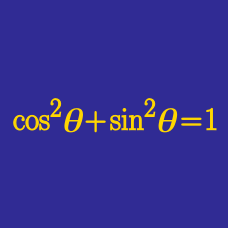Geometry

# Trigonometric Even-Odd Functions

Which of the following is equal to

$\sin ( - \theta) ?$

Which of the following is equal to

$\sec ( - \theta) ?$

Which of the following is equal to

$\cot ( - \theta) ?$

Which of the following is equal to

$\csc ( - \theta) ?$

Which of the following is equal to

$\tan ( - \theta) ?$

×